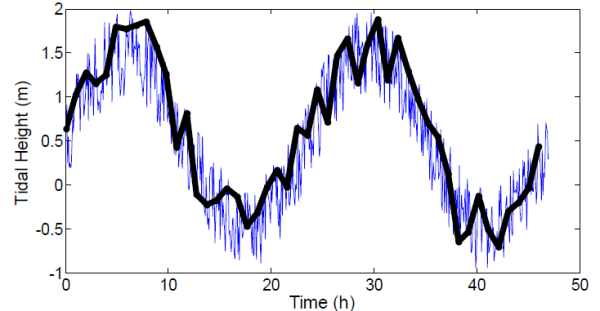## 1. 时间序列分析入门

### 避免Aliasing

Aliasing 通常是有害但又不可能100%避免的：

Nyquist Theorem

Anti-Aliasing Filters

RMS

RMS=root,mean,square. 用来描述信号质量。 计算方法: 一组数据，先平方，再求均值，最后开根。

``````function h=rms(data)
% h=rms(data)
% calculates root-mean-square
% of input matrix data
% Square the data using cell-by-cell multiplication
datasquared=data.*data;
% Calculate the mean of the squared data
mean_ds=mean(datasquared);
% Calculate the square-root of the mean
% h is what will be returned to the
% calling function
h=sqrt(mean_ds);``````

``rms(rand(1,1000))``

``````ans =

0.5649``````

## 2. FFT 傅里叶变换

fft和ifft(逆傅里叶变换)可以把信号进行时域和频域转换如下图。

``````srate = 100; % 100 Hz sample rate
speriod = 1/srate; % sample period (s)
dur=1; % duration in seconds
t=[0:speriod:dur-speriod];% Create a signal from 0 to 1 s. The sample period is 0.01 seconds.``````

``````freq=2; % frequency of sine wave in Hz
s=sin(2*pi*freq.*t); % calculate sine wave.
plot(t,s); % plot using t as the time base``````

``````sfft=fft(s); % calculate Fast Fourier Transform
sfft_mag=abs(sfft); % take abs to return the magnitude of the frequency components
plot(sfft_mag, '-.'); % plot fft result``````

``````fftpts=length(s);
hpts=fftpts/2; % half of the number points in FFT
sfft_mag_scaled=sfft_mag/hpts; % scale FFT result by half of the number of points used in the FFT
plot(sfft_mag_scaled); % plot scaled fft result``````

frequency resolution= sample rate / samples(points) in FFT

``````binwidth = srate/fftpts; % 100 Hz sample rate/ 100 points in FFT
f=[0:binwidth:srate-binwidth]; % frequency scale goes from 0 to sample rate.Since we are counting from zero, we subtract off one binwidth to get the correct number of points
plot(f,sfft_mag_scaled); % plot fft with frequency axis
xlabel('Frequency (Hz)'); % label x-axis
ylabel('Amplitude'); % label y-axis``````

``````%plot first half of data
plot(f(1:hpts),sfft_mag_scaled(1:hpts));
xlabel('Frequency (Hz)');
ylabel('Amplitude');``````

``[magnitude, index]=max(sfft_mag_scaled(1:hpts))``

Returns:

magnitude = 1

index = 3(从0开始，所以2Hz是3)

## 3. 数字滤波器

• FIR(有限冲击响应滤波器)
• IIR(无限冲击响应滤波器)

### FIR filter

``````npts=1000;
b=[.2 .2 .2 .2 .2]; % create filter coefficients for 5- point moving average

x=(rand(npts,1)*2)-1; % raw data from -1 to +1
filtered_data=filter(b,1,x); % filter using 'b' coefficients

subplot(2,1,1); % 1st subplot
plot(x); % plot raw data
title('Raw Data');
subplot(2,1,2); % 2nd subplot
plot(filtered_data); %plot filtered data
title('Filtered Data');
xlabel('Time')``````

End Bit 问题

filtered_data(2) = x(1)*0.2 + x(2)*0.2.

filtered_data(n) = b(1)*x(n) + b(2)*x(n-1) + b(3)*x(n-2) + b(4)*x(n-3) + b(5)*x(n-4).

``````% Perform FFT on original and filtered signal
fftpts=npts; % number points in FFT
hpts=fftpts/2; % half number of FFT points
x_fft=abs(fft(x))/hpts; %scaled FFT of original signal
filtered_fft=abs(fft(filtered_data))/hpts; %scaled FFT of filtered signal

subplot(2,1,1) %1st subplot
plot(x_fft(1:hpts)); %plot first half of data points
title('Raw Data');
subplot(2,1,2) %2nd subplot
plot(filtered_fft(1:hpts));%plot first half data points
title('Filtered Data');
xlabel('Frequency');``````

``````ntaps=20;
b=ones(1,ntaps)/ntaps; % create filter coefficients for ntap-point moving average``````

### IIR filter

IIR和FIR类似，不过进入了反馈机制。即下一次的滤波输出不仅仅和上几次的输入信号有关，还和上几次的输出信号有关。IIR比FIR"效率"更高，通常用更少的系数就可以达到很好的滤波结果，但是IIR也有缺点，由于引入了反馈机制，一些特定的系数组成的IIR滤波器可能不稳定，造成输出结果崩溃。。

``````% Butterworth IIR Filter
srate=1000; % sample rate
npts=2000; %npts in signal
Nyquist=srate/2; %Nyquist frequency
lpf=300; %low-pass frequency
order=5; %filter order
t=[0:npts-1]/srate; %time scale for plot
x=(rand(npts,1)*2)-1; % raw data from -1 to +1

[b,a]=butter(order,lpf/Nyquist); %create filter coefficients

filtered_data=filter(b,a,x); % filter using 'b' and 'a' coefficients

% Calculate FFT
fftpts=npts; % number points in FFT
hpts=fftpts/2; % half number of FFT points
binwidth=srate/fftpts;
f=[0:binwidth:srate-binwidth];
x_fft=abs(fft(x))/hpts; %scaled FFT of original signal
filtered_fft=abs(fft(filtered_data))/hpts; %scaled FFT of filtered signal

subplot(2,2,1)
plot(t,x);
title('Raw Time Series');
subplot(2,2,3)
plot(t,filtered_data);
title('Filtered Time Series');
xlabel('Time (s)');
subplot(2,2,2)
plot(f(1:hpts),x_fft(1:hpts));
title('Raw FFT');
subplot(2,2,4)
plot(f(1:hpts),filtered_fft(1:hpts));
title('Filtered FFT');
xlabel('Frequency (Hz)');``````

FDAtool

matlab有个神奇叫做 滤波器设计工具，以GUI的方式搞出你想要的滤波器。简直。。哎。没啥可说的，这玩意不要教程。。

IIR 滤波器原理

IIR 滤波器引入了'a' 系数反馈环节。 每一次滤波，上一次的输出也要程序对应的系数a 然后减到本次输出中：

y(n)=b(1)*x(n)+b(2)*x(n-1)+ ... + b(n)*x(n) - a(2)y(n-1) - a(3)*y(n-2)...

## 4. 自动信号检测

### 能量检测器

1. 对信号进行滤波，将有用信号提取出来
2. 对信号进行整流
3. 对整流过的信号进行包络
4. 设置阈值，检测有用信号

``````% Create Noise Signal and embed sine wave in it at a random location
npts=5000; % # points in signal
srate=1000; % sample rate (Hz)
dur=npts/srate; % signal duration in sec
amp_n=3; % noise amplitude
amp_t=1; % sine wave amplitude
freq=100; % sine wave frequency
sinepts=400; % # points in sine wave

t=[0:npts-1]/srate; % signal time base
sine_t=[0:sinepts-1]/srate; % sine wave time base
noise=(rand(1,npts)-.5)*amp_n; %noise signal
sinewave=amp_t*(sin(2*pi*freq*sine_t)); % sine wave
% random location of sinewave
sinepos=floor(rand(1,1)*(npts-sinepts))+1;
signal = noise; % make signal equal to noise
endsine = sinepos + sinepts-1; %calc index end of sine wave
% add sinewave to signal starting at index sinepos
signal(sinepos:endsine) = signal(sinepos:endsine) + sinewave;

% Plot signal
subplot(5,1,1)
plot(t,signal);
hold on
%plot red dot on location of sine wave start
plot(sinepos/srate,0,'.r');
hold off
title('Original Signal');

``````

step1: 对信号进行滤波, 将有用信号提取出来， 我们搞一个 带宽为10Hz的 带通滤波器，中心频点设置在需要提取的正弦信号位置，这一波操作代码如下：

``````% Step 1: Filter signal
hbandwidth=5; %half bandwidth
nyquist=srate/2;
ntaps=200;
hpf=(freq-hbandwidth)/nyquist;
lpf=(freq+hbandwidth)/nyquist;
[b,a]=fir1(ntaps, [hpf lpf]); %calc filter coefficients
signal_f=filter(b,a,signal); %filter signal
subplot(5,1,2)
plot(t,signal_f); %plot filtered signal
hold on
%plot red dot on location of sine wave
plot(sinepos/srate,0,'.r');
hold off
title('Step 1. Filter Signal');``````

step2: 整流信号，其实就是取绝对值，把负的信号弄成正的:

``````signal_r=abs(signal_f); %abs value of filtered signal
subplot(5,1,3)
plot(t,signal_r); %plot filtered signal
hold on
%plot red dot on location of sine wave
plot(sinepos/srate,0,'.r');
hold off
title('Step 2. Rectify Signal');``````

step3: 对整流过的信号进行包络。 求整流过的信号的包络。可以通过一个低通滤波器实现。这里我们用一个6极点butter 滤波器实现，截止频率是 10Hz。过了这个滤波器，滤波后的信号基本就成了原信号的包络。注意信号的起始位置，因为过了滤波器，所有可以看出一点点的相位滞后

``````% Step 3: Low-pass filter rectified signal
lpf=10/nyquist; % low-pass filter corner frequency
npoles=6;
[b,a]=butter(npoles, lpf); % Butterworth filter coeff
signal_env=filter(b,a,signal_r); %Filter signal
subplot(5,1,4)
plot(t,signal_env); %plot filtered signal
hold on
%plot red dot on location of sine wave
plot(sinepos/srate,0,'.r');
hold off
title('Step 3. Envelope Signal');``````

step4: 给信号设置阈值，搞一个变量gated, 当原信号超过一个阈值的时候，gated=1, 否则=0

``````% Step 4: Threshold signal
threshold=amp_t/2;
gated=(signal_env>threshold);
subplot(5,1,5)
plot(t,signal_env); %plot filtered signal
hold on
%plot red dot on location of sine wave
plot(sinepos/srate,0,'.r');
plot(t, gated, 'r'); % plot threshold signal
hold off
title('Step 4. Threshold Signal');
xlabel('Time (s)');``````

setp5： diff一把，找到信号

``````d_gated=diff(gated);
plot(d_gated);``````

## 5. 图像处理

``````rows=20; % variable for # rows
stripes=5;% variable for # stripes
% make one column of 1's and an adjacent column of 0's
x=horzcat(ones(rows,1),zeros(rows,1));
y=[]; %initialize and clear y matrix
for n=1:stripes
y=horzcat(y,x);% concatenate x onto y
end
clim=[0 1]; % color limits for imagesc
imagesc(y, clim); % plot scaled image
colormap(gray); % use gray scale color map``````

### 读取和操作图像

matlab读取和操作图像很简单

``````r=imread('reef.jpg','jpg');
image(r)``````

``````r(1,1,1:3)
ans(:,:,1) =
66
ans(:,:,2) =
153
ans(:,:,3) =
174``````

step1: 把蓝色分量取出来，绘制灰度图

``````r=imread('reef.jpg','jpg');
%mage(r)

rb= r(:,:,3);
imagesc(rb);
colormap(gray);``````

``````rbt=rb<130; %threshold dark values
imagesc(rbt)
colormap(gray)``````

``````% calculate sum of all white points (=1)
reef=sum(sum(rbt)); %reef pts
totalpts=prod(size(rbt)); %#pts in image
percentreef=reef/totalpts``````

## 附录 - DFT(离散傅里叶变换)

• X(m) 是变换的输出X(0), X(1),X(2)...X(N-1). m是输出频域上的频率值. m = 0,1,2,3,4...N-1
• x(n)是输入信号x(0), x(1), x(2)... n就是时域上的坐标。。
• N就是输入 sample的数量

FFT输出的第一个点为：